Definitions of Square Dance Calls and Concepts

Index -->  Plus  |  A1  |  A2  |  C1  |  C2  |  C3A  |  C3B  |  C4  |  NOL  |
Definitions (Text Only) -->  Plus  |  A1  |  A2  |  C1  |  C2  |  C3A  |  C3B  |  C4  |  NOL  |
 Find call:

\$BG\$0U\$N(B 4 \$B?M\$N(B line \$B\$^\$?\$OB>\$NE,@Z\$J(B formation \$B\$+\$i!%(B

1. Centers Cross Run;

4 \$B?M\$N(B line \$B\$+\$i\$O(B 4 \$B?M\$N(B line \$B\$G=*\$o\$j\$^\$9!%(B

Wave \$B\$+\$i(B: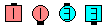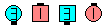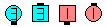Mix\$B\$NA0(B Centers Cross Run\$B\$N8e(B Centers Trade\$B\$N8e(B (\$B=*\$o\$j(B)
One-Faced Line \$B\$+\$i(B: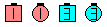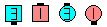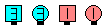Mix\$B\$NA0(B Centers Cross Run\$B\$N8e(B Centers Trade\$B\$N8e(B (\$B=*\$o\$j(B)
T-Bone 2 x 4 \$B\$+\$i(B: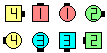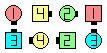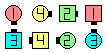Mix\$B\$NA0(B Centers Cross Run\$B\$N8e(B Centers Trade\$B\$N8e(B (\$B=*\$o\$j(B)

\$BCm
• \$BA40w4i\$N8~\$-\$r(B 180°\$BJQ\$(\$^\$9(B.
• Centers \$B\$O(B, \$B>/\$7A0?J\$7\$F\$+\$i(B Cross Run \$B\$r\$9\$Y\$-\$G\$9!%(B \$B\$3\$l\$O(B ends \$B\$,(B center \$B\$NJ}\$X2#\$KF~\$k>l=j\$r\$"\$1\$F(B, \$BF0\$-\$d\$9\$/\$9\$k\$?\$a\$G\$9!%(B
• \$B\$b\$7(B centers \$B\$,F1\$8J}\$r8~\$\$\$F\$\$\$k\$H\$3\$m\$+\$i;O\$a\$k\$H\$-\$O(B, centers \$B\$O\$*8_\$\$\$K(B \$B:88*(B \$B\$GDL\$j2a\$.\$^\$9!%(BCenters \$B\$O(B Cross Run (Half Sashay and Run) \$B\$r\$9\$k\$+\$i\$G\$9!%(B
• \$B%*%j%8%J%k\$N(B ends \$B\$O(B \$B8~\$-\$rJQ\$(\$:\$K(B centers \$B\$XF~\$j(B Trade \$B\$r\$9\$Y\$-\$G\$9!%(B \$BF~\$k\$H\$-\$K8~\$-\$rJQ\$(\$F\$7\$^\$&\$H(B, \$B\$b\$&(B1\$B?M\$r:.Mp\$5\$;\$F\$7\$^\$\$(B Trade \$B\$,\$-\$A\$s\$H\$G\$-\$J\$/\$J\$j\$^\$9!%(B \$B\$b\$&(B1\$B?M\$N(B ends \$B\$K\$H\$C\$F(B, \$B\$"\$J\$?\$N%*%j%8%J%k\$N8~\$-\$,J,\$+\$i\$J\$/\$J\$j(B, mini wave \$B\$+\$i(B Arm Turn 1/2 \$B\$r\$9\$k\$N\$+(B, \$B%+%C%W%k\$+\$i(B Partner Trade \$B\$r\$9\$k\$N\$+\$,J,\$+\$i\$J\$/\$J\$j:.Mp\$7\$^\$9!%(B
•  Mix \$B\$O(B Diamond \$B\$+\$i\$G\$-\$^\$9\$+(B? \$B\$\$\$\$\$(!%(BCenters \$B\$K\$H\$C\$F2s\$kAjl=j\$K\$O(B \$B@8?H\$N%@%s%5!<(B \$B\$,\$\$\$J\$1\$l\$P\$J\$j\$^\$;\$s(B)\$B!%(B C1 \$B\$G\$O(B, Squeeze The Diamond [Centers \$B\$O(B Trade & Spread; \$B\$=\$7\$F%*%j%8%J%k\$N(B Ends \$B\$,6aIU\$\$\$F(B Trade] \$B\$,(B, diamond \$B\$+\$i\$N(B Mix \$B\$GK>\$^\$l\$?7k2L\$rF@\$k\$?\$a\$NBe\$o\$j\$H\$7\$F;H\$(\$k\$G\$7\$g\$&!%(B

Swing & Mix [A2] (Lee Kopman 1967): \$B8~\$+\$\$9g\$C\$?%+%C%W%k(B, wave, inverted line \$B\$+\$i(B, Arm Turn 1/2 - Centers Cross Run - (new) Centers Trade \$B\$r\$7\$^\$9!%(B Wave \$B\$^\$?\$O8~\$+\$\$9g\$C\$?%+%C%W%k\$+\$i\$O(B wave \$B\$G=*\$o\$j(B, inverted line \$B\$+\$i\$O(B inverted line \$B\$G=*\$o\$j\$^\$9!%(B 3 \$B%Q!<%H\$N%3!<%k\$G\$9!%(B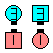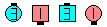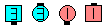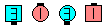Swing & Mix\$B\$NA0(B Arm Turn 1/2\$B\$N8e(B Centers Cross Run\$B\$N8e(B (new) Centers Trade\$B\$N8e(B (\$B=*\$o\$j(B)

\$BCm
• \$B\$=\$7\$F8e\$K(B anything & Mix \$B\$H0lHL2=\$5\$l\$^\$7\$?!%(B
• Swing & Mix is no longer explicitly listed on the CALLERLAB A2 list. However, the calls Swing [A2] and Mix [A1] are on CALLERLAB lists. In our opinion, Swing & Mix should be listed on the A2 list as a separate call because it can be done from Facing Couples. According to the current CALLERLAB definitions, a Swing & Mix is a Swing followed by a Mix. Swing, as defined by CALLERLAB, must start from a Wave or Inverted Line, not Facing Couples. Swing is defined as Ends and Adjacent Centers Arm Turn 1/2. However, Swing & Mix is still widely called from Facing Couples, and it should be legal.
• Swing & Mix \$B\$O(B, \$BNr;KE*\$JM}M3\$K\$h\$j(B 3 \$B%Q!<%H\$N%3!<%k\$G\$9!%(B
• 1/4 (or 3/4) Mix [C3A]: \$B1&\$N(B mini wave box, centers \$B\$,1&\$N(B mini wave \$B\$N(B diamond, \$B\$^\$?\$OB>\$NE,@Z\$J(B formation \$B\$+\$i!%(B \$B\$G\$-\$k?M\$,(B Right Arm Turn 1/4 (\$B\$^\$?\$O(B 3/4) \$B\$r\$7(B, line|wave \$B\$G(B Mix \$B\$r\$7\$^\$9!%(B Line \$B\$^\$?\$O(B wave \$B\$G=*\$o\$j\$^\$9!%(B3 \$B%Q!<%H\$N%3!<%k\$G\$9!%(B

Grand Mix [C3A]: \$B0lHLE*\$J(B tidal line \$B\$+\$i!%(B Center \$B\$N%Z%!(B (8\$B?M(B \$B\$N(B tidal line \$B\$+\$i\$J\$i(B3\$B%Z%!(B, 6\$B?M(B \$B\$N(B tidal line \$B\$+\$i\$J\$i(B2\$B%Z%!(B) \$B\$,(B, Grand (Working As Centers) Cross Run (Grand Slip-Slide \$B\$N\$h\$&\$K(B) \$B\$r\$7(B, \$B?7\$7\$\$(B center \$B\$N%Z%!\$,(B Trade (Grand Slip) \$B\$r\$7\$^\$9!%(B Tidal line \$B\$G=*\$o\$j\$^\$9!%(B 2 \$B%Q!<%H\$N%3!<%k\$G\$9!%(BChoreography for MixComments? Questions? Suggestions?

https://www.ceder.net/def/mix.php?language=japan
16-September-2021 03:02:10